# FORMULATEXT Function

Returns a formula in text form from the referred cell

## What is the FORMULATEXT Function?

The FORMULATEXT Function helps you get a formula in text form from the referred cell. FORMULATEXT is categorized under Excel Lookup and Reference functions. It was introduced in MS Excel 2013.

### Formula

=FORMULATEXT(reference)

Reference here is the reference to a cell or range of cells from which we want the formula as text.

### How to use the FORMULATEXT Function in Excel

It is a built-in function which can be used as a worksheet function in Excel. Let’s take an example.

Let’s assume we receive a file from a co-worker who used different formulas for different cells. The workbook comprises 20 worksheets and around 100 formulas are used in those 20 sheets. To keep track of the formulas used and ensure errors are avoided, we can use the FORMULATEXT function.

As shown below, the worksheet includes data on sales and quantity sold. The user used different formulas in column F. Using the FORMULATEXT function, we can get the formulas used in cells.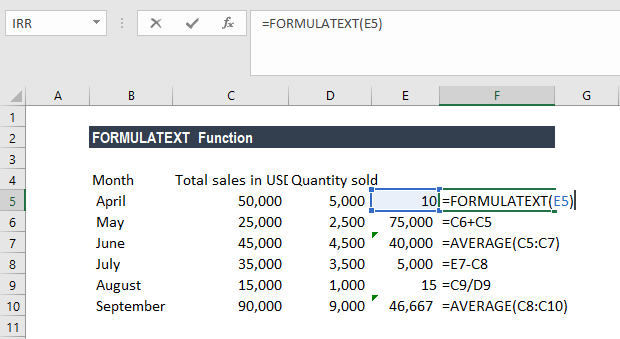When we drag down this formula, it will show us the formulas used in column F. In this manner, instead of using F2 and checking each and every formula, we now see it in the adjacent column.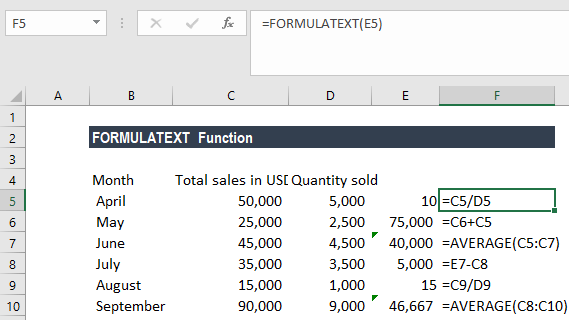### Examples of the FORMULATEXT Function

To understand the uses of this function, let’s consider a few examples:

#### Example 1

Let’s consider the example below, using the Total Sales and Net Income data for different years.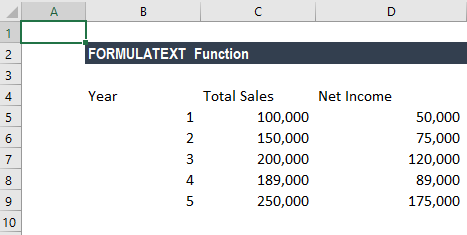As shown below, we’ve estimated the Sales and Income for the current year. The estimates are based on the assumption that sales would be the average of the last 5 years plus 10% of that Average, and Income would be 65% of the Estimated Sales.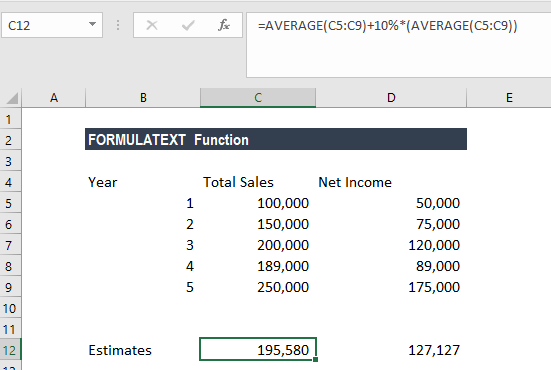Now if we wish to document this, FORMULATEXT can be used to document all the formulas in the workbook. The function becomes very useful when we are using a workbook with lots of data and formulas and helps in reducing errors.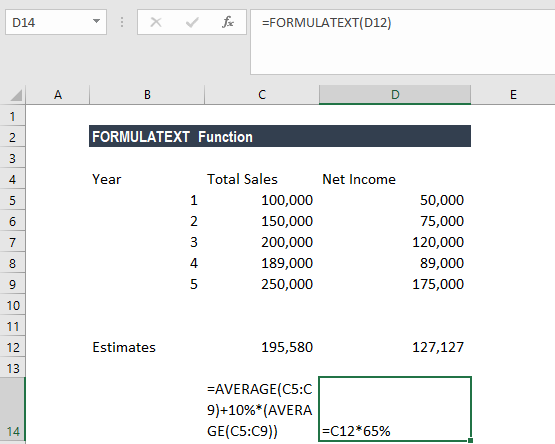#### Example 2

Even if we enter a reference to a cell in which the FORMULATEXT function was entered as an argument, still the circular reference warning will not be returned and the function will return the formula as text in the cell.

Let’s continue with same data that was used in Example 1. So, when I give a reference of D14 in which we used the FORMULATEXT function, still I will not get a circular reference warning. Instead, we shall get the formula as text as shown below: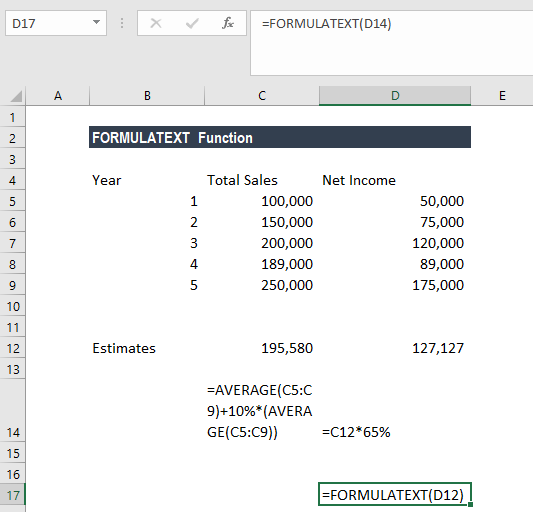As we can see above, FORMULATEXT gave us the formula used in D14 instead of a circular reference.

### A few pointers on the FORMULATEXT function

• #N/A error occurs when:

1.  We have used the function and referred a cell that doesn’t have any formula on it.

2.  We have referred it to another workbook and that workbook is not open.

3.  When the formula in the cell is too long. The function supports only 8192 characters.

4.  If the worksheet cannot be opened as it is a protected worksheet.

• #VALUE! error occurs when an invalid data type is used as input.
• Suppose we are using an Excel worksheet with lots of data and formulas. Now we aren’t sure whether a formula was used in all rows of, let’s say, column D. In this scenario, we can use ISFORMULA to find out the same and then apply FORMULATEXT function.
• We entered too many arguments. This error would pop up when we give reference to more than one cell.
• If the reference given in the function is for more than one cell, it will give us the formula that is in the upper left cell of the given range.
• If we are using older versions of Excel, we need to use VBA to get the Formula used in the cell.

Thanks for reading CFI’s guide to important Excel functions! By taking the time to learn and master these functions, you’ll significantly speed up your financial analysis. To learn more, check out these additional CFI resources:

• Excel Functions for Finance